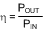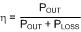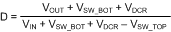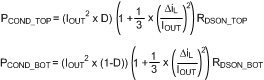JAJSAY9L February   2009  – May 2018

PRODUCTION DATA.

1. 特長
2. アプリケーション
3. 概要
1.     Device Images
4. 改訂履歴
5. Pin Configuration and Functions
6. Specifications
7. Detailed Description
1. 7.1 Overview
2. 7.2 Functional Block Diagram
3. 7.3 Feature Description
4. 7.4 Device Functional Modes
8. Application and Implementation
1. 8.1 Application Information
2. 8.2 Typical Applications
1. 8.2.1 LM26420X 2.2-MHz, 0.8-V Typical High-Efficiency Application Circuit
2. 8.2.2 LM26420X 2.2-MHz, 1.8-V Typical High-Efficiency Application Circuit
3. 8.2.3 LM26420X 2.2-MHz, 2.5-V Typical High-Efficiency Application Circuit
4. 8.2.4 LM26420Y 550 kHz, 0.8-V Typical High-Efficiency Application Circuit
5. 8.2.5 LM26420Y 550-kHz, 1.8-V Typical High-Efficiency Application Circuit
6. 8.2.6 LM26420Y 550-kHz, 2.5-V Typical High-Efficiency Application Circuit
9. Power Supply Recommendations
10. 10Layout
11. 11デバイスおよびドキュメントのサポート
1. 11.1 デバイス・サポート
2. 11.2 ドキュメントのサポート
3. 11.3 ドキュメントの更新通知を受け取る方法
4. 11.4 コミュニティ・リソース
5. 11.5 商標
6. 11.6 静電気放電に関する注意事項
7. 11.7 Glossary
12. 12メカニカル、パッケージ、および注文情報

• PWP|20
• RUM|16
• PWP|20
• RUM|16

#### 8.2.1.2.5 Calculating Efficiency and Junction Temperature

The complete LM26420 DC/DC converter efficiency can be estimated in the following manner.

Equation 17.Or

Equation 18.Calculations for determining the most significant power losses follow here. Other losses totaling less than 2% are not discussed.

Power loss (PLOSS) is the sum of two basic types of losses in the converter: switching and conduction. Conduction losses usually dominate at higher output loads, whereas switching losses remain relatively fixed and dominate at lower output loads. The first step in determining the losses is to calculate the duty cycle (D):

Equation 19.VSW_TOP is the voltage drop across the internal PFET when it is on, and is equal to:

Equation 20. VSW_TOP = IOUT × RDSON_TOP

VSW_BOT is the voltage drop across the internal NFET when it is on, and is equal to:

Equation 21. VSW_BOT = IOUT × RDSON_BOT

If the voltage drop across the inductor (VDCR) is accounted for, the equation becomes:

Equation 22.Another significant external power loss is the conduction loss in the output inductor. The equation can be simplified to:

Equation 23. PIND = IOUT2 × RDCR

The LM26420 conduction loss is mainly associated with the two internal FETs:

Equation 24.If the inductor ripple current is fairly small, the conduction losses can be simplified to:

Equation 25. PCOND_TOP = (IOUT2 × RDSON_TOP × D)
Equation 26. PCOND_BOT = (IOUT2 × RDSON_BOT × (1-D))
Equation 27. PCOND = PCOND_TOP + PCOND_BOT

Switching losses are also associated with the internal FETs. They occur during the switch on and off transition periods, where voltages and currents overlap resulting in power loss. The simplest means to determine this loss is to empirically measuring the rise and fall times (10% to 90%) of the switch at the switch node.

Switching Power Loss is calculated as follows:

Equation 28. PSWR = 1/2(VIN × IOUT × FSW × TRISE)
Equation 29. PSWF = 1/2(VIN × IOUT × FSW × TFALL)
Equation 30. PSW = PSWR + PSWF

Another loss is the power required for operation of the internal circuitry:

Equation 31. PQ = IQ × VIN

IQ is the quiescent operating current, and is typically around 8.4 mA (IQVINC = 4.7 mA + IQVIND = 3.7 mA) for the 550-kHz frequency option.

Due to Dead-Time-Control Logic in the converter, there is a small delay (~4 nsec) between the turn ON and OFF of the TOP and BOTTOM FET. During this time, the body diode of the BOTTOM FET is conducting with a voltage drop of VBDIODE (~0.65 V). This allows the inductor current to circulate to the output, until the BOTTOM FET is turned ON and the inductor current passes through the FET. There is a small amount of power loss due to this body diode conducting and it can be calculated as follows:

Equation 32. PBDIODE = 2 × (VBDIODE × IOUT × FSW × TBDIODE)

Typical Application power losses are:

Equation 33. PLOSS = ΣPCOND + PSW + PBDIODE + PIND + PQ
Equation 34. PINTERNAL = ΣPCOND + PSW+ PBDIODE + PQ

### Table 3. Power Loss Tabulation

DESIGN PARAMETER VALUE DESIGN PARAMETER VALUE
VIN 5 V VOUT 1.2 V
IOUT 2 A POUT 2.4 W
FSW 550 kHz
VBDIODE 0.65 V PBDIODE 5.7 mW
IQ 8.4 mA PQ 42 mW
TRISE 1.5 nsec PSWR 4.1 mW
TFALL 1.5 nsec PSWF 4.1 mW
RDSON_TOP 75 mΩ PCOND_TOP 81 mW
RDSON_BOT 55 mΩ PCOND_BOT 167 mW
INDDCR 20 mΩ PIND 80 mW
D 0.262 PLOSS 384 mW
η 86.2% PINTERNAL 304 mW

These calculations assume a junction temperature of 25°C. The RDSON values are larger due to internal heating; therefore, the internal power loss (PINTERNAL) must be first calculated to estimate the rise in junction temperature.